Function Repository Resource:

Write a quadratic expression as a sum of squares by eliminating its mixed terms and then completing squares

Contributed by: Dennis M Schneider
 ResourceFunction["DiagonalizeQuadratic"][quad,vars,newvars] returns the diagonalized quadratic in newvars, the eigenvalues, corresponding orthonormal eigenvectors and a list of substitution rules relating newvars to vars.

## Details and Options

In the diagonalized quadratic that is returned, the new variables are related to the original variables by the equation newvars=vars. Transpose[m] where the columns of the matrix m are the eigenvectors of quad. The coefficients of the squared terms are the eigenvalues.

## Examples

### Basic Examples (3)

Rewrite a quadratic with no linear terms:

 In:=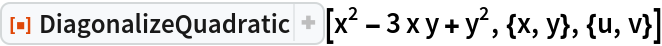Out=Confirm the result:

 In:=Out=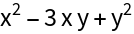Rewrite a quadratic with linear terms:

 In:=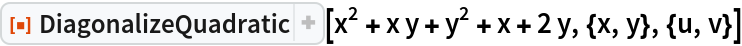Out=Confirm the result:

 In:=Out=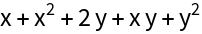Rewrite a quadratic in three variables:

 In:=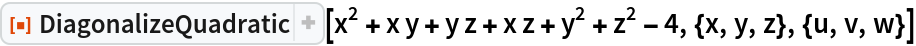Out=Confirm the result:

 In:=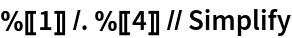Out=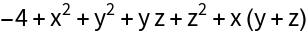### Scope (2)

When Mathematica returns Root objects in determining the eigensystem of the matrix, DiagonalizeQuadratic returns numerical approximations of the eigenvalues and eigenvectors:

 In:=Out=Check the result:

 In:=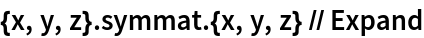Out=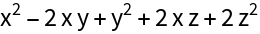Mathematica returns numerical approximations for the eigensystem of symmat:

 In:=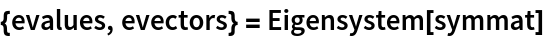Out=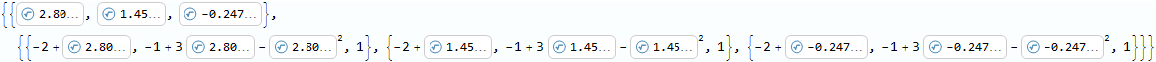In:=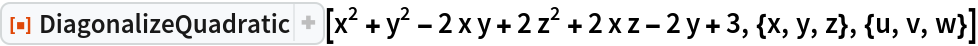Out=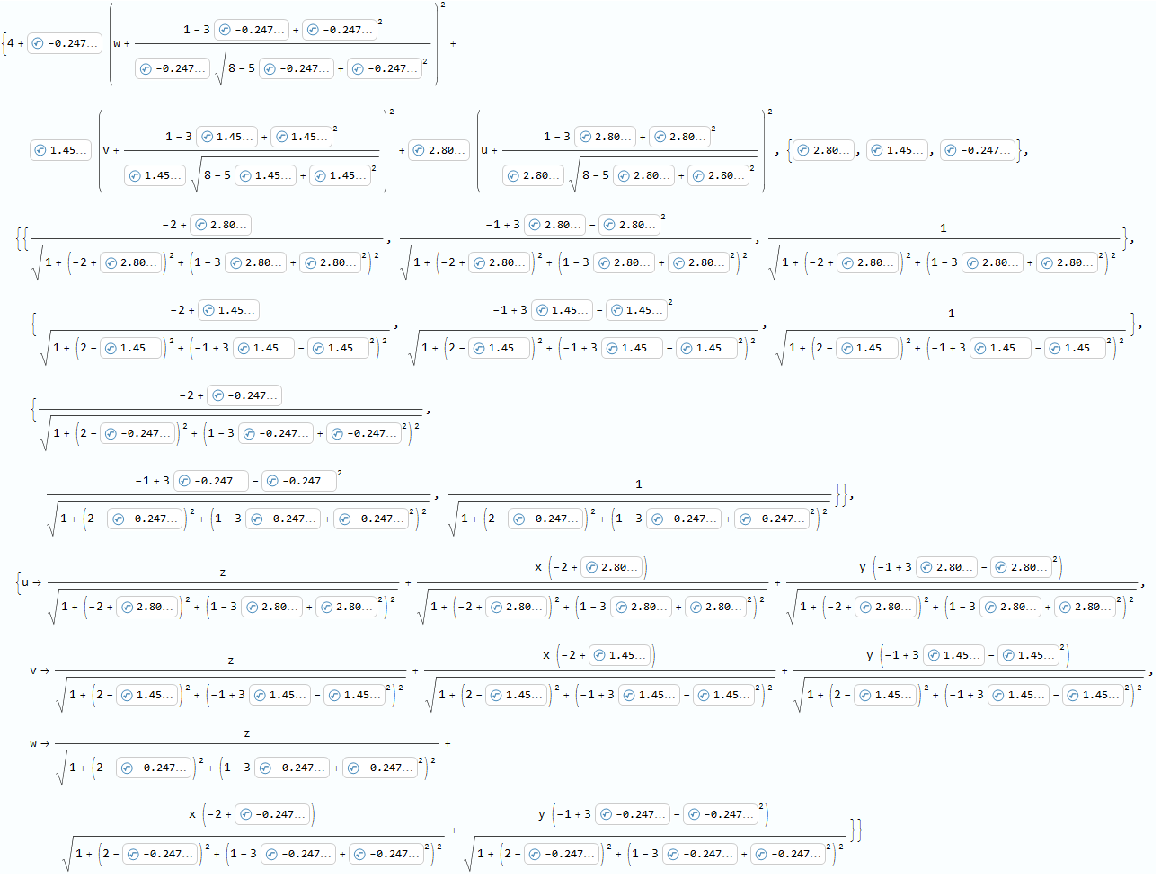In:=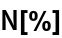Out=The input can be given as an equation:

 In:=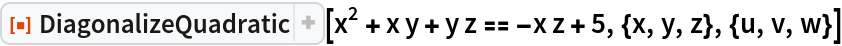Out=### Possible Issues (4)

The names of the original variables and the new variables must be disjoint:

 In:=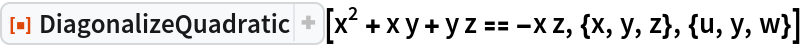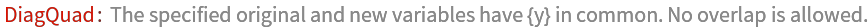The list of variables and new variables must have the same length:

 In:=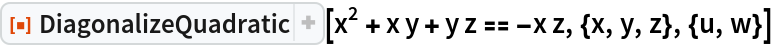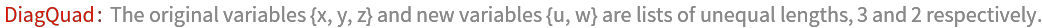If new variables are not specified, an error message is returned:

 In:=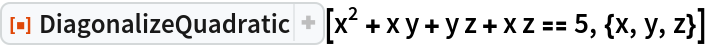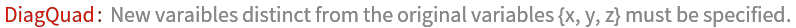Note that if the input quadratic is numericized by applying N to it, then the diagonalized quadratic returned is different from the diagonalized quadratic returned by diagonalizing the non-numericized quadratic. The reason is that Mathematica's Eigensystem returns a different (but equivalent) list of orthonormal eigenvectors:

 In:=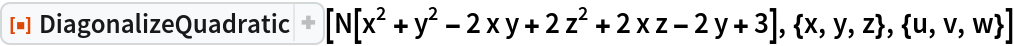Out=This output is different from the previous output:

 In:=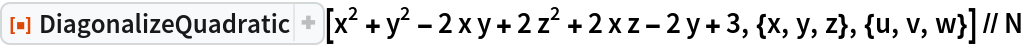Out=In:=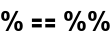Out=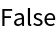## Publisher

Dennis M Schneider

## Version History

• 2.0.0 – 12 September 2019
• 1.0.0 – 16 August 2019